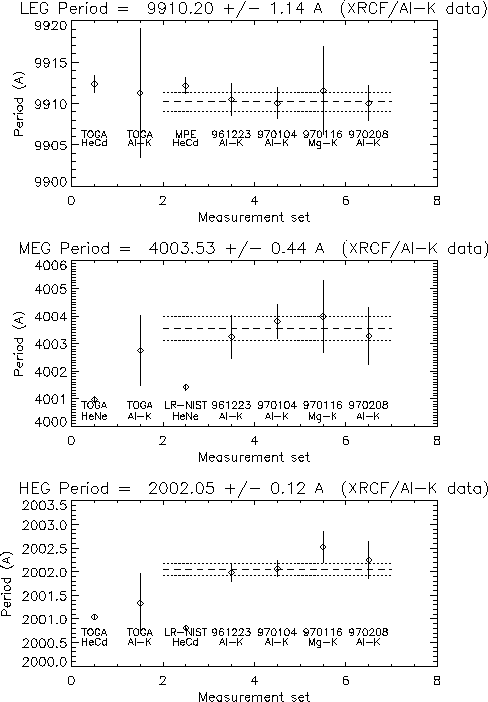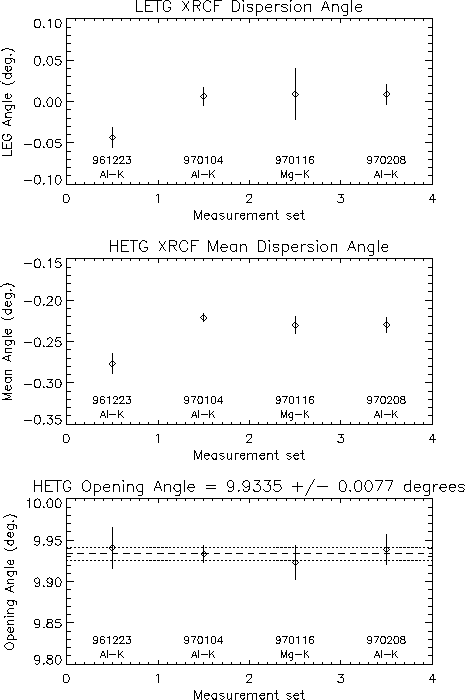Period (and angle) Analysis

## Period (and angle) Analysis

Go to HETG at XRCF

### Results and Discussion

(Updated 9/17/97 dd)

The results to date suggest adopting the following calibration values:

GratingAverage PerioderrorXRCF Rowland Spacingerror
LEG9912.16+/- 0.998788.04+/- 0.5
MEG4001.41+/- 0.108782.8+/- 0.5
HEG2000.81+/- 0.058782.8+/- 0.5
GratingXRCF mean AngleerrorOpening Angleerror
LETG+0.010+/- 0.05N/AN/A
HETG-0.225+/- 0.059.934+/- 0.008

The periods (in A) are the sub-assembly values because XRCF measurements (and spacing) are not accurate (double checked) enough to require a change. The mean dispersion angles are for XRCF and are a good starting point for flight; the HETG opening angle should be the same for XRCF and flight.

The HEG/MEG period difference seen between sub-assembly (HeCd,HeNe) and XRCF-AL-K (assuming a spacing of 8788.04)(of about a part in 500) is assumed to be due to a mis-placing of the HETG at XRCF. Thus, for now the HETG position at XRCF is set to be 8782.8 mm to have reasonable agreement between XRCF and sub-assembly periods. The cause for the (alleged) displacement has not been determined.

The period errors are all taken as the sub-assembly values, again because (with an HETG displacement assumption) XRCF measurements are in agreement and do not provide any smaller error bounds.

The XRCF Rowland Spacing, 8788.04 +/- 0.5 mm is based on data supplied by Scott Texter (TRW) in a diagram dated 8/15/97. The HETG and LETG were each measured to be within 0.05 mm of this mean spacing value. (HETG = 345.984 inches, LETG = 345.986 inches). This value differs by 5.24 mm from the value implied by design documentation (see Location of HETG at XRCF).

### Flight Grating Spacing

Scott Texter reports that EK measures the as-installed HETG to be 0.009 inches closer to the HRMA than designed, so expected flight spacing is 8633.69+0.009*25.4 = 8633.92 mm. The error on this is probably 0.2 mm or less (HRMA-grating spacing). However the HRMA-focus spacing may still have error of order 0.5 mm. Likewise, the as-installed LETG distance is 8633.69 - 0.0043*25.4 = 8633.58 mm.

### Analysis chain

• Lab measurements were made on the flight gratings for LETG and HETG as well as on the TOGA gratings used in XRCF rehearsal. These laboratory measurements were corrected based on NIST-measured reference samples resulting in our best estimate "LR-NIST" periods.
• At XRCF Encircled Energy measurements of the diffracted orders were performed with HXDS Beam Centering in order to precisely locate the diffracted images.
• The beam centers obtained from these measurements are put into data files:
• Analyze each of these data files with xrcf_beam_cen_werr.pro to get a best-fit period and angle (with errors) for each grating. Put the results, along with sub-assembly predicted values, into the results file:
• Plot the Period and Angle contents of this results file using beam_cen_plots.pro. Calculate the weighted-average period for each grating based only on the XRCF Al-K data sets. Calculate the HETG opening angle.

### To-do

• Improve analysis of EE data
• Add mst_date and run_id values to 961223 and 970104 data files.
• Re-do full analysis: .pha files to .sum files
• Plot .sum files, compare with expected 1D scans (simulations); defocus?
• Better "center" location and errors using 1D simulated shapes
• ( Explain the LETG / HETG difference in spacing )
• Is the TOGA MEG period difference due to old LR version used for TOGA?
• Why the HEG Al/Mg difference? ( by 1 part in 4000 )
• Use HSI observations for period determination too.
• Use HRC and ACIS-S data sets to determine period/angle as well.
• Is 8782.8 mm the best value for spacing?

• Get some flight data and compare to emission line predictions!

### Plots of XRCF Period Determination Results

Assumptions: Rowland distance = 8788.04 mm, hc = 12.3985, Al-K is at 1.4867 keV. TOGA rowland distance = 5366.55 mm.